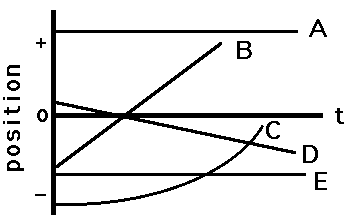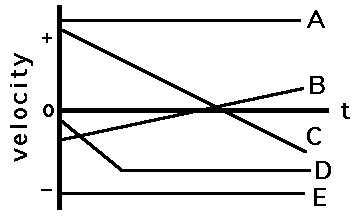# Graph Sketching and Recognition

The following practice questions test your understanding of the graphical description of motion. Use the Show Answer! button to view answers and explanations. Further information is available on-line at The Physics Classroom Tutorial.

NOTE to the Student:

## Use the following graph to answer Questions #1 - #7.1. Which object(s) is(are) maintaining a state of motion (i.e., maintaining a constant velocity)?

2. Which object(s) is(are) accelerating?

3. Which object(s) is(are) not moving?

4. Which object(s) change(s) its direction?

5. On average, which object is traveling fastest?

6. On average, which moving object is traveling slowest?

7. Which object has the greatest acceleration?

## Use the following graph to answer Questions #8 - #13.8. Which object(s) is(are) maintaining its state of motion?

9. Which object(s) is(are) accelerating?

10. Which object(s) is(are) not moving?

11. Which object(s) change(s) its direction?

12. Which accelerating object has the smallest acceleration?

13. Which object has the greatest velocity?

14. Sketch a position-time graph for an object which is moving with a constant, positive velocity.

15. Sketch a position-time graph for an object which is moving with a constant, negative velocity.

16. Sketch a position-time graph for an object moving in the + dir'n and accelerating from a low velocity to a high velocity.

17. Sketch a position-time graph for an object moving in the + dir'n and accelerating from a high velocity to a low velocity.

18. Sketch a position-time graph for an object moving in the - dir'n and accelerating from a high velocity to a low velocity.

19. Sketch a position-time graph for an object moving in the - dir'n and accelerating from a low velocity to a high velocity.

20. Sketch a position-time graph for an object moving in the + dir'n with constant speed; first a slow constant speed and then a fast constant speed.

21. Sketch a position-time graph for an object moving in the + dir'n with constant speed; first a fast constant speed and then a slow constant speed.

22. Sketch a position-time graph for an object moving in the - dir'n with constant speed; first a slow constant speed and then a fast constant speed.

23. Sketch a position-time graph for an object moving in the - dir'n with constant speed; first a fast constant speed and then a slow constant speed.

24. Sketch a position-time graph for an object which moves in the + direction at a slow constant speed and then in a - direction at a fast constant speed.

25. Sketch a position-time graph for an object which moves in the + direction at a fast constant speed and then in a - direction at a slow constant speed.

26. Sketch a position-time graph for an object which moves in the - direction at a slow constant speed and then in a + direction at a fast constant speed.

27. Sketch a velocity-time graph for an object moving with a constant speed in the positive direction.

28. Sketch a velocity-time graph for an object moving with a constant speed in the negative direction.

29. Sketch a velocity-time graph for an object which is at rest.

30. Sketch a velocity-time graph for an object moving in the + direction, accelerating from a slow speed to a fast speed.

31. Sketch a velocity-time graph for an object moving in the + direction, accelerating from a fast speed to a slow speed.

32. Sketch a velocity-time graph for an object moving in the - direction, accelerating from a slow speed to a fast speed.

33. Sketch a velocity-time graph for an object moving in the - direction, accelerating from a fast speed to a slow speed.

34. Sketch a velocity-time graph for an object which first moves with a slow, constant speed in the + direction, and then with a fast constant speed in the + direction.